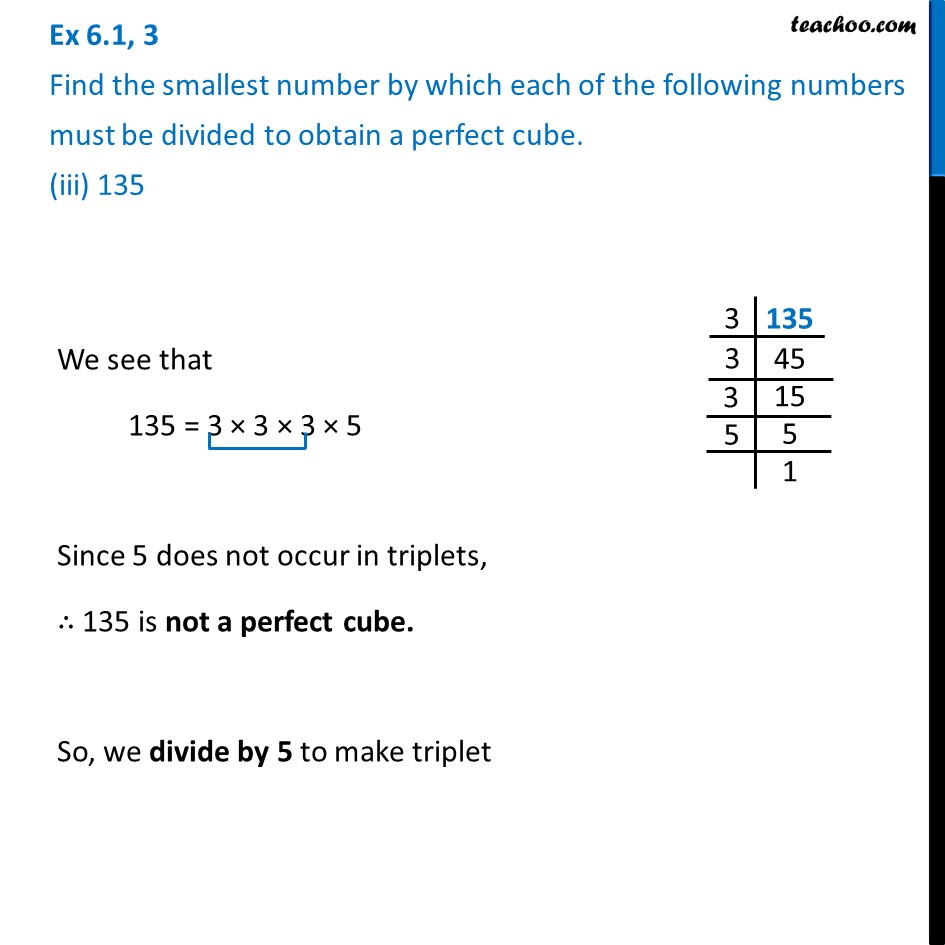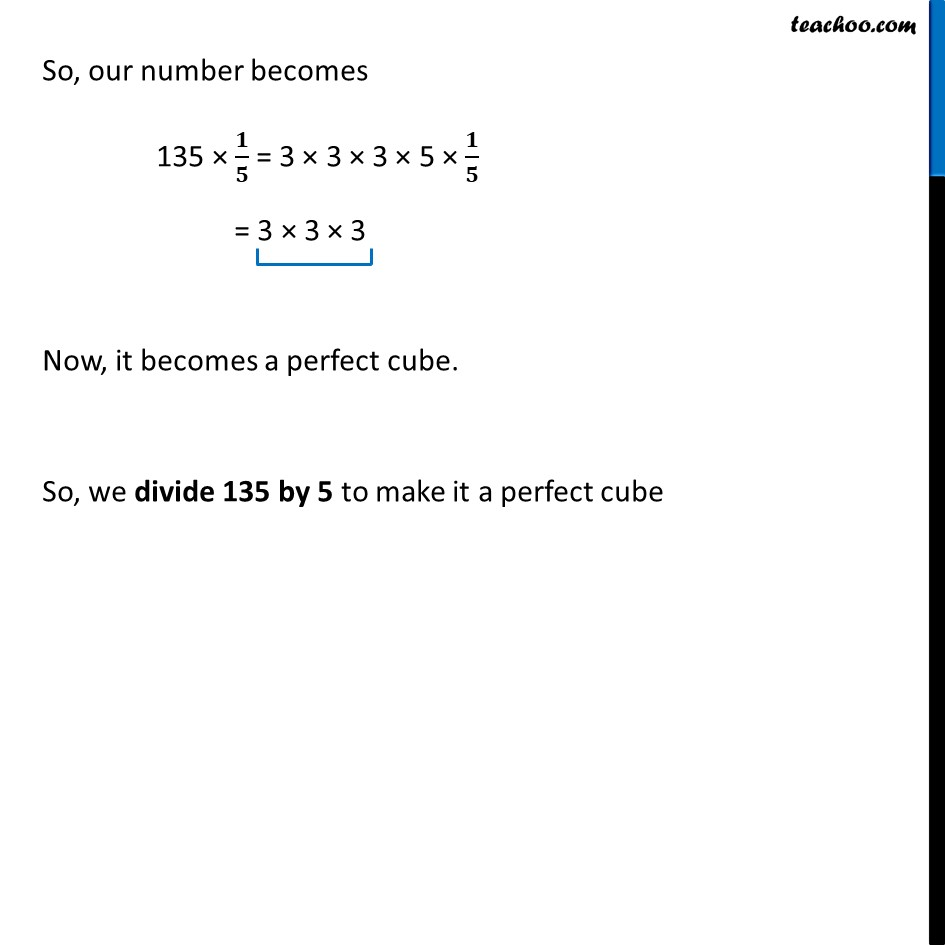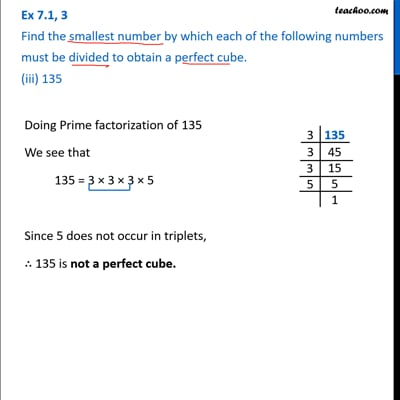Ex 6.1

Chapter 6 Class 8 Cubes and Cube Roots
Serial order wiseThis video is only available for Teachoo black users

Learn in your speed, with individual attention - Teachoo Maths 1-on-1 Class

### Transcript

Ex 6.1, 3 Find the smallest number by which each of the following numbers must be divided to obtain a perfect cube. (iii) 135 We see that 135 = 3 × 3 × 3 × 5 Since 5 does not occur in triplets, ∴ 135 is not a perfect cube. So, we divide by 5 to make triplet So, our number becomes 135 × 𝟏/𝟓 = 3 × 3 × 3 × 5 × 𝟏/𝟓 = 3 × 3 × 3 Now, it becomes a perfect cube. So, we divide 135 by 5 to make it a perfect cube